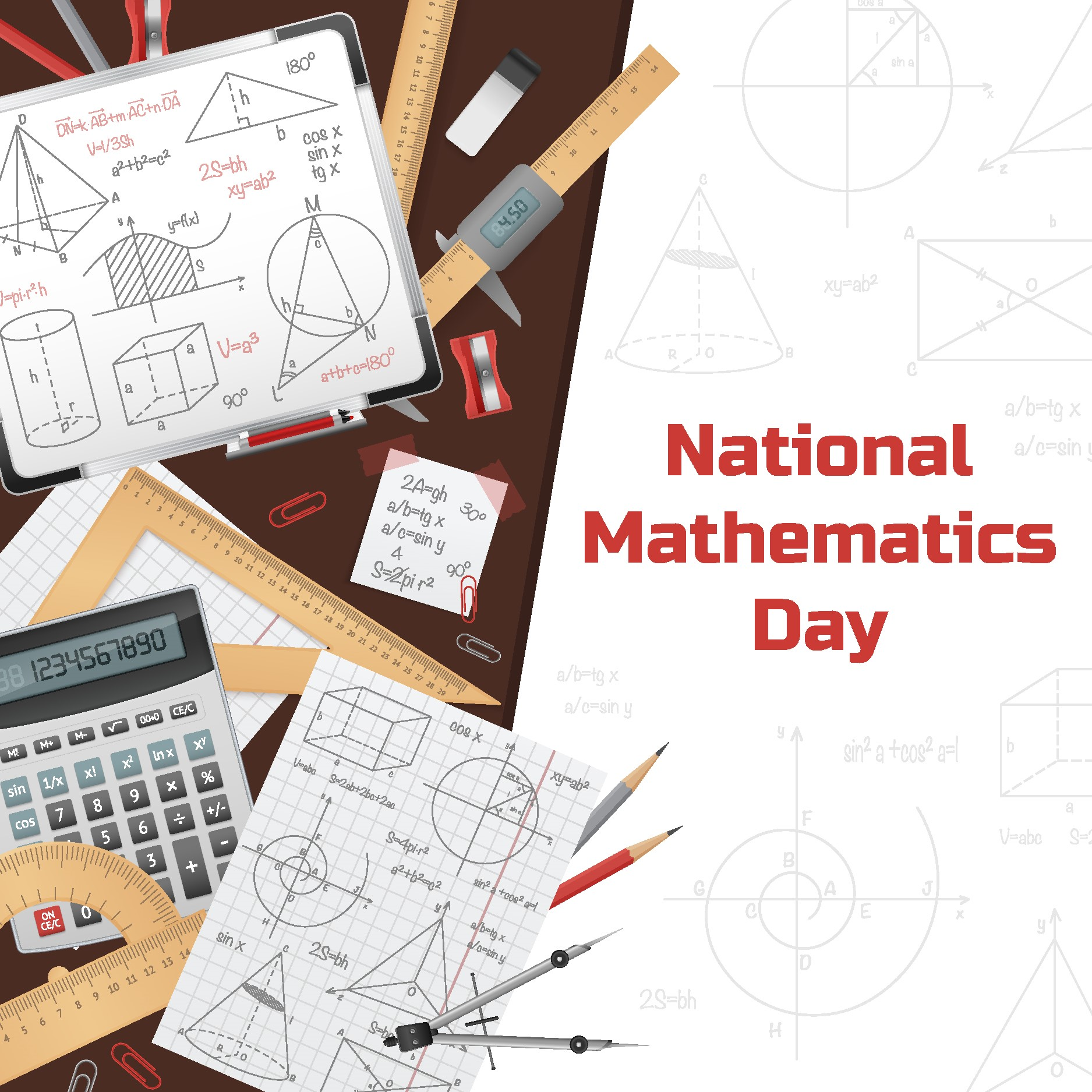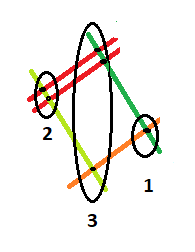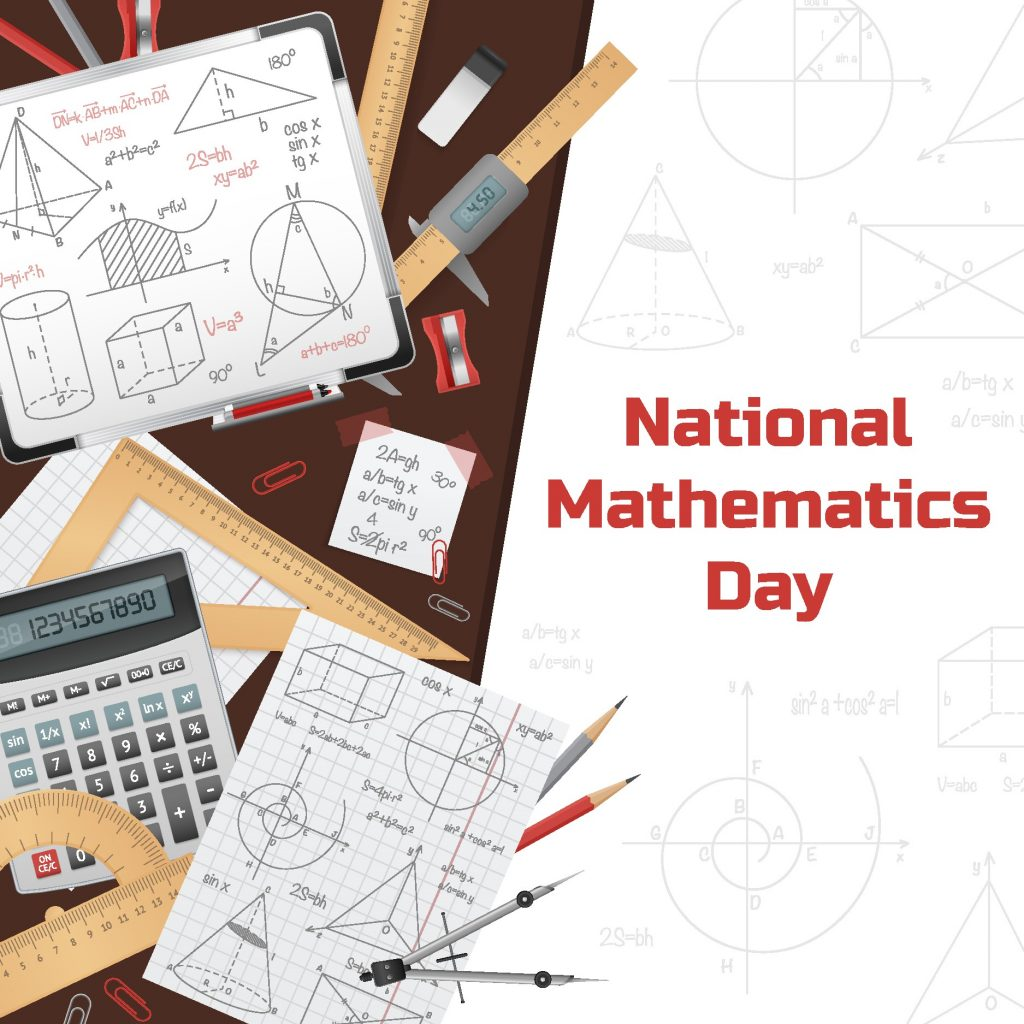# National Mathematics DayNational Mathematics Day

WHAT AND WHY?

22nd December celebrates the birth anniversary of an Indian mathematician Srinivasa Ramanujan as ‘National Mathematics Day’. In 2012 Manmohan Singh, the former Prime Minister of India had declared his birthday as National Mathematics Day because he is a reckon among pioneers in Mathematics.

Mathematics is simply the study of numbers, shape and patterns.

The word came from a Greek word “mathema” meaning science knowledge and learning.

Mathematics is often considered to be difficult.  Around 80% of students are likely to dislike mathematics as they find it difficult. Many people came up with tips to make mathematics  interesting with some clever tricks to solve it.

Let’s review them and learn some tricks to save our time and solve complicated calculations with ease.

• The Japanese Chopstick trick:

Mathematics axis was set years before pen and paper or any technical device came into existence.

At that point of time the Japanese people used chopsticks to solve it. Let’s see how they actually do it.

Multiplication of 2 digit numbers.

Let’s have a look at it step wise.

Step 1- Draw lines corresponding to the number. Firstly, for the first set of numbers.

For example- your number is 21 (draw two lines together with a little space and one line parallel to the two lines with and after a bigger space)

Step 2- Draw lines for the other set of numbers in the same way from left to right but diagonally.

(By now the diagram should roughly look like a diamond shape)

Step 3 – Now count the points where the lines intersect each other under the diamond.

Step 4- Start adding the intersecting points from the left to right.

Step 5- Write  the numbers you get in a sequence and you get your final result.

The same steps can be followed for more digits numbers.

Let’s try one: Suppose we are multiplying 21 x 11 (Don’t touch the calculator!!!)So the answer is 231!!! (Now you can check!!)

Without doing a single sum and taking much time you can solve a difficult sum.

This can be useful during exams when you aren’t allowed technical devices.

Note: This is called chopsticks method because earlier instead of pen and paper the Japanese people used chopsticks to make the lines and solve the multiplication.

• Vedic Mathematics:

The science mathematics in an easier and faster way Jagadguru Sri Bharati Krishna Tirthayi has compiled various tricks and techniques in a book called the Vedic Mathematics.

These have been used since the ancient period to solve various calculations especially by the Indian scientist and mathematicians.

Some of them are listed below :

Note: These tricks are easy and can be carried out in simple steps.

Let’s get introduced to some of them and make mathematics fun, easier and interesting.

• Multiplying two three digit numbers-

Let’s take 996 and 998 as an example.

Step 1- Both the numbers are closer to 10 power (base is 1000)

Step 2- 996 is 4 less than 1000 and 998 is 2 less than 1000.

Step 3- (4) *(2 ) = 8 (since base is 1000 take 008)

Step 4- 996-2 or 998-4= 994

So, the final answer should be 994008.

• Calculating square value (easy and fast trick)

Let’s do it with an example to understand it better.

We take a number 99

Step 1- choose a base closer to the original number.

Here, the base would be 100

Step 2- find the difference of the number from the base

99-100= – 1

Step 3- add the difference with the original number

99+(-1) = 98

Step 4 – multiply the result with base

98 ×100 = 9800

Step 5= now, add result with the square of the difference

9800 + (-1)² = 9801

So the final result is  9801

People have always tend to find shortcuts and tricks to solve problems.The above mentioned tricks are useful and applicable.

Besides Ramanujan, there are other Indian mathematicians who contributed to the development of mathematics and made it better. Who discovered various things.

One of them is Aryabhatta who discovered the digit Zero and made a commendable significance.

Shakuntala Devi is a renowned writer and she is famous for her extraordinary calculating and numerical skills.

Mathematics can be fun and boring to people as per their interest.

But as stated “Pure mathematics is, in its way, the poetry of logical ideas”

— Albert Einstein.

Everything around us is numbers and understanding numbers is ultimately mathematics.

Have passion to learn everything and it will never be boring as now I know the amazing ideas and tricks to solve them.

-Ester Pariyar

## Related Post# National Mathematics Day